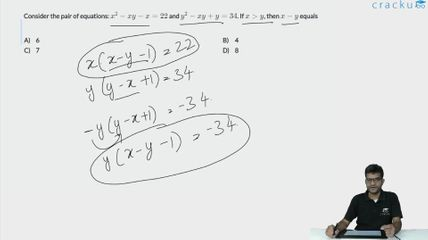Question 63

# Consider the pair of equations: $$x^{2}-xy-x=22$$ and $$y^{2}-xy+y=34$$. If $$x>y$$, then $$x-y$$ equals

Solution

We have :
$$x^2-xy-x\ =22\ \ \ \ \ \ \left(1\right)$$
And $$y^2-xy+y\ =34\ \ \ \ \ \ \left(2\right)$$
we get $$x^2-2xy+y^2-x+y\ =56$$
we get $$\left(x-y\right)^2-\ \left(x-y\right)\ =56$$
Let (x-y) =t
we get $$t^2-t=56$$
$$t^2-t-56=0$$
(t-8)(t+7) =0
so t=8
so x-y =8

### View Video Solution